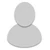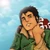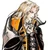## FANDOM

26,104 Pages

We are going to figure out how much energy it really takes to bust mountains of different sizes.

For a minimum size of a mountain to have Mountain level yield at minimum via:

### Fragmentation: Mountain level

• (4.184e17 Joules) / (8 Joules/cc) = 5.230e16 cm^3, or 5.230e10 m^3 destroyed.
• Assuming that a mountain is considered a cone, the volume for that is (Pi)*(Radius^2)*(Height/3)
• Assuming that the mountain's height and radius are equal, the minimum values for both radius and height should be about 3682.55m.
• Plug the above number for both radius and height for the cone volume and you get the volume required for Mountain level via fragmenting it.

### Violent Fragmentation: Mountain level

• (4.184e17 Joules) / (69 Joules/cc) = 6.064e15 cm^3, or 6.064e9 m^3 destroyed.
• Assuming that a mountain is considered a cone, the volume for that is (Pi)*(Radius^2)*(Height/3)
• Assuming that the mountain's height and radius are equal, the minimum values for both radius and height should be about 1795.72m.
• Plug the above number for both radius and height for the cone volume and you get the volume required for Mountain level via violent fragmentation.

### Pulverization: Mountain level

• (4.184e17 Joules) / (214 Joules/cc) = 1.955e15 cm^3, or 1.955e9 m^3 destroyed.
• Assuming that a mountain is considered a cone, the volume for that is (Pi)*(Radius^2)*(Height/3)
• Assuming that the mountain's height and radius are equal, the minimum values for both radius and height should be about 1231.33m.
• Plug the above number for both radius and height for the cone volume and you get the volume required for Mountain level via pulverization.

### Vaporization: Mountain level

• (4.184e17 Joules) / (25700 Joules/cc) = 1.547e13 cm^3, or 1.547e7 m^3 destroyed.
• Assuming that a mountain is considered a cone, the volume for that is (Pi)*(Radius^2)*(Height/3)
• Assuming that the mountain's height and radius are equal, the minimum values for both radius and height should be about 249.16m.
• Plug the above number for both radius and height for the cone volume and you get the volume required for Mountain level via vaporization.

For a minimum size of a mountain to have Large Mountain level yield at minimum via:

### Fragmentation: Large Mountain level

• (4.184e18 Joules) / (8 Joules/cc) = 5.230e17 cm^3, or 5.230e11 m^3 destroyed.
• Assuming that a mountain is considered a cone, the volume for that is (Pi)*(Radius^2)*(Height/3)
• Assuming that the mountain's height and radius are equal, the minimum values for both radius and height should be about 7934m.
• Plug the above number for both radius and height for the cone volume and you get the volume required for Large Mountain level via fragmenting it.

### Violent Fragmentation: Large Mountain level

• (4.184e18 Joules) / (69 Joules/cc) = 6.064e16 cm^3, or 6.064e10 m^3 destroyed.
• Assuming that a mountain is considered a cone, the volume for that is (Pi)*(Radius^2)*(Height/3)
• Assuming that the mountain's height and radius are equal, the minimum values for both radius and height should be about 3868.76m.
• Plug the above number for both radius and height for the cone volume and you get the volume required for Large Mountain level via violent fragmentation.

### Pulverization: Large Mountain level

• (4.184e18 Joules) / (214 Joules/cc) = 1.955e16 cm^3, or 1.955e10 m^3 destroyed.
• Assuming that a mountain is considered a cone, the volume for that is (Pi)*(Radius^2)*(Height/3)
• Assuming that the mountain's height and radius are equal, the minimum values for both radius and height should be about 2652.81m.
• Plug the above number for both radius and height for the cone volume and you get the volume required for Large Mountain level via pulverization.

### Vaporization: Large Mountain level

• (4.184e18 Joules) / (27050 Joules/cc) = 1.547e14 cm^3, or 1.547e8 m^3 destroyed.
• Assuming that a mountain is considered a cone, the volume for that is (Pi)*(Radius^2)*(Height/3)
• Assuming that the mountain's height and radius are equal, the minimum values for both radius and height should be about 528.63m.
• Plug the above number for both radius and height for the cone volume and you get the volume required for Large Mountain level via vaporization.

Usually, an island is going to be shaped like a circle. For example, a typical island will look like this:

For a minimum size of an island to have Island level yield at minimum via:

### Fragmentation: Island level

• (1.8e19 Joules) / (8 Joules/cc) = 2.250e18 cm^3, or 2.25e12 m^3 destroyed.
• Mean/Average height of land elevation above sea level is 840m (Google)
• Volume of rectangle = Length*Width*Height, where Height = 840m
• Volume of cylinder = (Pi)*(Radius^2)*(Height), where Height = 840m
• Isolate for both Length and Width, assuming that both are equal, and you get a value of 51755m for length and width of said island respectively.

If the island is Rectangular:

• The dimensions of said island would have to be 51755m x 51755m x 840m to yield Island level values fragmenting it.

If the island is Cylindrical, the dimensions of said island would have to be:

• Height = 840m

in order to yield Island level values fragmenting it.

### Violent Fragmentaton: Island level

• (1.8e19 Joules) / (69 Joules/cc) = 2.61e17 cm^3, or 2.61e11 m^3 destroyed.
• Mean/Average height of land elevation above sea level is 840m
• Volume of rectangle = Length*Width*Height, where Height = 840m
• Volume of cylinder = (Pi)*(Radius^2)*(Height)
• Isolate for both Length and Width, assuming that both are equal, and you get a value of 5574m for length and width of said island respectively.

If the island is Rectangular:

• The dimensions of said island would have to be 17627m x 17627m x 840m to yield Island level value via violent fragmentation.

If the island is Cylindrical, the dimensions of said island would have to be:

• Height = 840m

in order to yield Island level values via violent fragmentation.

### Pulverization: Island level

• (1.8e19 Joules) / (214 Joules/cc) = 8.411e16 cm^3, or 8.411e10 m^3 destroyed.
• Mean/Average height of land elevation above sea level is 840m
• Volume of rectangle = Length*Width*Height, where Height = 840m
• Volume of cylinder = (Pi)*(Radius^2)*(Height)
• Isolate for both Length and Width, assuming that both are equal, and you get a value of 5574m for length and width of said island respectively.

If the island is Rectangular:

• The dimensions of said island would have to be 10007m x 10007m x 840m to yield Island level value via violent fragmentation.

If the island is Cylindrical, the dimensions of said island would have to be:

• Height = 840m

in order to yield Island level values via pulverization.

### Vaporization: Island level

• (1.8e19 Joules) / (27050 Joules/cc) = 6.654e14 cm^3, or 6.654e8 m^3 destroyed.
• Mean/Average height of land elevation above sea level is 840m
• Volume of rectangle = Length*Width*Height, where Height = 840m
• Volume of cylinder = (Pi)*(Radius^2)*(Height)
• Isolate for both Length and Width, assuming that both are equal, and you get a value of 5574m for length and width of said island respectively.

If the island is Rectangular:

• The dimensions of said island would have to be 17627m x 17627m x 840m to yield Island level value via vaporization.

If the island is Cylindrical:

• Well, apparently the radius of said island is less than the height; it's unrealistic for an island to have these dimensions, so we'll skip this part.

If we are talking about explosions, the minimum surface area that an explosion needs to cover to make Mountain/Large Mountain/Island level should be about (We will use Near-Total Fatalities for this):

### Mountain level Explosion:

• Radius Near Total Fatalities = 15.7km
• Surface Area = 7.74e8 m^2. The explosion needs to cover this much circular area to make Mountain level.

### Large Mountain level Explosion:

• Radius Near Total Fatalities = 33.6km
• Surface Area = 3.55e9 m^2. The explosion needs to cover this much circular area to make Large Mountain level.

### Island level Explosion:

• Radius Near Total Fatalities = 54.4km
• Surface Area = 9.30e9 m^2. The explosion needs to cover this much circular area to make Island level.

Credit to ThePerpetual for the next part.

Continuing from the above article from earlier, we will now be discussing the energy required to destroy mountains of differing size.

For this, we will look at two mountains, each of varying shape and size, as well as an island.

• A small mountain (A minimum size mountain)
• A large mountain (Mount Fuji)
• An Island (Cyprus Island)

1) A small mountain

• Height = 517px, or 609.6m
• Radius = Width/2 = 1849px/2 = 924.5px, or 1090.1m
• Volume = (Pi)*(Radius^2)*(Height/3) = (3.1416)*(1090.1^2)*(609.6/3) = 758590275.6m^3, or 7.586e14 cm^3.

To destroy it:

• Fragmentation (8 J/cc) = 6.070e15 Joules, Small City level
• Violent Fragmentation (69 J/cc) = 5.234e16 Joules, City level
• Pulverization (214 J/cc) = 1.623e17 Joules, or City level
• Vaporization (27050 J/cc) = 2.052e19 Joules, or Island level

2) Mount Fuji

• Height = 130px = 3776m
• Radius = Width/2 = 708px/2 = 354px = 10282.3m
• Volume = (Pi)*(Radius^2)*(Height/3) = (3.1416)*(10282.3^2)*(3776/3) = 4.181e11 m^3, or 4.181e17 cm^3

To destroy it:

• Fragmentation (8 J/cc) = 3.345e18 Joules, Mountain level+
• Violent Fragmentation (69 J/cc) = 2.885e19 Joules, Island level
• Pulverization (214 J/cc) = 8.947e19 Joules, Island level
• Vaporization (27050 J/cc) = 3.345e22 Joules, Small Country level

3) Cyprus Island

• Surface Area = 9251km^2 (Check "Area")
• Radius = sqrt(Area/(Pi)) = Sqrt(9251000000m^2/(3.1416)) = 54265m, or ~54.265km

To destroy it:

• Near-Total Fatalities = 8.6e3 Megatons of TNT, or 8.6e9 Tons of TNT.
• Divide the actual yield by 2, as only 50% of the energy of explosion is released as the actual explosion, thus we get a yield of 4300 Megatons of TNT, or 4.3 Gigatons of TNT. Island level

## What Have We Learned?

• It takes around Small City level to City level amount of energy to destroy a small mountain, depending on how it is destroyed.
• It takes around Large Mountain level to Island level to destroy a large mountain, depending on how it is destroyed.
• Vaporizing an actual mountain requires a surprisingly large amount of energy, considering that it takes a massive amount of energy to turn actual rock into steam.
• It takes 4.3 Gigatons of TNT to destroy Cyprus Island.

## Start a Discussion Discussions about Mountain and Island level requirements

•#### Master Yi Upgrade to 7-A - Mountain Slashing Feat.

4 messages
•bump.
•This is not the right time for new content revision threads. My apologies. https://vsbattles.fandom.com/wiki/Thread:4342423
•#### Mount Everest AP question

4 messages
•It depends on how everest was destroyed.
•High 6-C if you vaporized it and High 7-A if you pulverized it I believe.
Community content is available under CC-BY-SA unless otherwise noted.